## 4.3 MOND

The interpretation of the rotation curve of spiral galaxies is based on the assumption that Newtonian Dynamics is valid. This assumption is not accepted by the so called "Modified Newtonian Dynamics" (MOND) developed and discussed by Milgrom (1983a, b, c), Sanders(1990) and others. An implicit rule in our approach to Cosmology is that our physical laws are valid everywhere, unless they lead us to unacceptable conclusions. This law is therefore apparently violated. But Newtonian Dynamics was established after considering nearby astronomical phenomena, and we are allowed to modify it now, when we are aware of distant large-scale phenomena, unknown in Newton's time. Moreover, MOND aims not only to explain the rotation curve of spirals, but to propose an alternative theory of Gravitation and/or Dynamics. With respect to galaxies, the introduction of MOND provides very remarkable fits, rendering this theory a very interesting alternative.

As an introduction, let us consider the outermost disk, where the galactic mass can be considered a central point producing a gravitational potential that predicts a Keplerian decrease. But instead of the standard form for the acceleration of gravity, g = GM/r2, assume that it is expressed as K(M)/r, where K(M) is a constant depending only on the galactic mass. If this force is matched by the centrifugal force,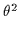/r, we readily obtain= K(M), therefore obtaining that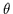is a constant and thus the rotation curve paradox is automatically solved, without the need for dark matter, at least for bright galaxies.

What would be the dependence of K on M? A good assumption in the absence of dark matter could be that the M/L ratio is independent of R. Therefore, if K(M) = xM1/2 with x being any constant, then we would directly obtain M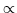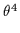, and therefore, L, which is the Tully-Fisher relation. Thus, we have already solved the two basic problems of the rotation of spirals: flat rotation curves and the Tully-Fisher relation. Therefore, g would depend on M1/2, or equivalently, on (GM)1/2. Hence, g=constant (GM)1/2R-1. The constant would have the dimensions of the square root of an acceleration and would be a universal constant. Let us call this acceleration a0. Finally, g = (GMa0)1/2R-1. The constant a0 was introduced by Milgrom (1983a).

Let us estimate its value. If this kind of gravity is supported by rotation,= GMa0. Taking200kms-1 and M = 1011M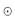, as typical values in a spiral (without dark matter), we obtain a01.2 × 10-8cms-2. More precise estimations provide 2 × 10-8cms-2 (Milgrom, 1983b, for H0 = 50kms-1Mpc-1) or 1.2 × 10-8cms-2 (Begeman et al. 1991, with H0 = 75).

But we know that g = GM/R2 for familiar dynamical systems. It could be that the standard expression is valid for small R, and the tested expression (GMa0)1/2R-1 is valid for large R. In the whole region g could obey the sum of the two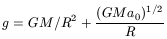(84)

the first term would predominate at low R, and the second at large R. This expression was first proposed by Sanders (1990).

The transition region would be characterized by a similar order of magnitude of the two terms, i.e. for R(transition) around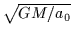. For M of the order of a galactic mass, the transition would take place at around 10 kpc (but a galaxy is not a central point mass), thus suggesting that at large radii, the new gravitational term would predominate, which solves both the flat rotation curve and the Tully-Fisher problems.

This equation is just a first simple example to see how our problems can be solved by modifying Newton's Universal Law of Gravitation. There is a second way, consisting in accepting this law, but modifying Newton's Second Law,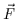= m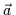. Which procedure is the best? Apparently, the first one is preferable, as the whole of Newtonian Dynamics remains valid and we just modify a law that was proposed by Newton from the observations, but without claiming it to be a fundamental principle. In the same way, Einstein's Field Equations could be modified without rejecting General Relativity. Therefore, modifying the gravitational law would be a much softer procedure than modifying the Second Law. However, for gravitational purposes, both procedures are equivalent.

Let us reconsider the problem from a more general point of view.

In MOND, Milgrom (1983a) proposed, instead of the Second Law, that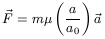(85)

with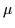(x) being a function to be determined, of which we only know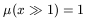(86)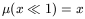(87)

i.e., for low accelerations, much less than a0, the Second Law would be substituted by= m(a/a0), being the force proportional to the squared acceleration. In this way, in a galaxy, with a =/R we would have GM/R2 = (/R2)a0 again solving both the flat rotation problem and the Tully-Fisher relation.

Alternatively, the modification of the gravitational law could be expressed in a general form as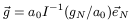(88)

where I is an unknown function, and therefore its inverse, I-1, is also an unknown function; gN is the standard Newtonian gravitational acceleration and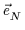is a unit vector with the standard direction of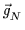.

The directions of all vectors are the same, and therefore we can denote our derivations without vector arrows. If gN is the classical Newton gravitational acceleration we rewrite (85) as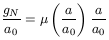(89)

The arguments of both functionsand I-1 are any variable, but we will keep the notation gN/a0 = u and a/a0 = x, therefore, instead of (89)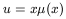(90)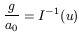(91)

(91) is equivalent to modifying the gravitational force while retaining Newton's Second Law, g = a, therefore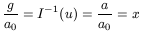(92)

hence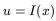(93)

With (90)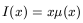(94)

If x1,(x) = x, hence I(x) = x2, I-1(u) = u1/2.

If x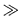1,(x) = 1, hence I(x) = x, I-1(u) = u.

We have stated that the modification of Newton's Second Law, while retaining the expression of the gravitational force, is equivalent to the modification of the gravitational force and retaining Newton's Second Law. We will show this with two examples, based on the above expressions.

The first example considers the modification of Newton's Second Law, in the way first proposed by Milgrom (1983b), corresponding to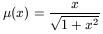(95)

This expression is interesting as it is the simplest form of accomplishing our asymptotic conditions (86) and (87). With (95) Newton's Second Law would be replaced by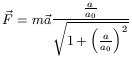(96)

which for aa0, effectively reduces to= m.

Then our question becomes what is the equivalent transformation of the gravitational force, i.e. producing the same dynamical effects as the simple proposal of Milgrom (1983b) in (95)? We just need to find I, and then I-1 can be inserted in (88). With (94)(97)

With (93)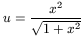(98)

hence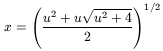(99)

The signus (-) provides an non physical solution. With (92)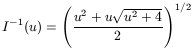(100)

Observe that if u1, then I-1(u) = u, which means that in regions where Newton's classical gravitational force is high ( gNa0), the gravitational force coincides with the classical one. But, if u1, I-1(u) = u1/2 and g = (a0gN)1/2, it is rather different. The complete expression of g would become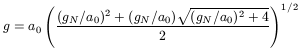(101)

an interesting formula because it is obtained without modification of the three general laws of Newtonian Mechanics, explains flat rotation curves without dark matter and explains the Tully-Fisher relation.

As a second example, suppose the inverse problem. We start with a modification of Newtonian gravitational force and want to know the equivalent modification of Newton's Second Law. We must first propose a new form of the gravitational force. We suppose that (86) is the correct expression as we have seen that it also explains flat curves and the Tully-Fisher relation. First, we rewrite (86) as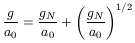(102)

With (88) and u = gN/a0, this is written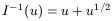(103)

or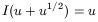(104)

With (93)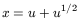(105)

or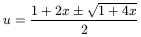(106)

With (93) again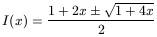(107)

With (94)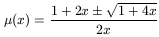(108)

If x1,(x) = 1, which matches (86). If x1,(x) =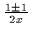. If we take the signus (+), we have(x) = x-1 which is not correct, following (87). We then take the signus (-); then we apparently obtain(x) = 0. But we should then expand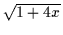1 + 2x - 2x2 and therefore(x) = x in agreement with (87). The signus (-) therefore gives us the physical solution. The complete modified Second Law would then be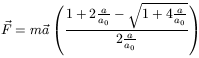(109)

which could be a general expression, Newton's Second Law could be just an approximation for high accelerations.

Subsections